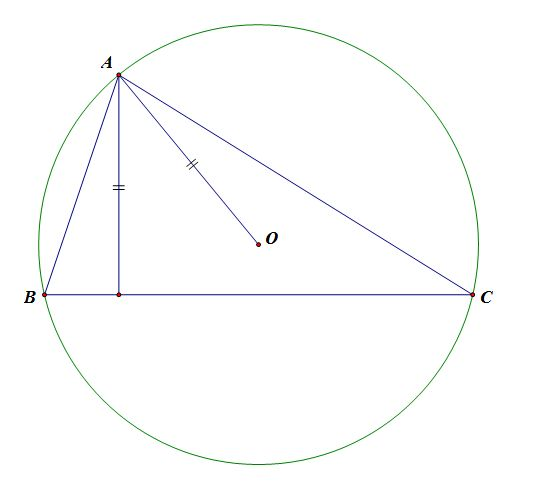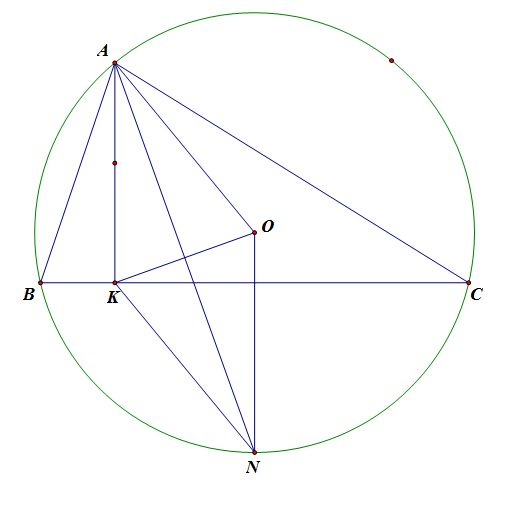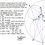# What happens when $R=h_A$?(Basics of Construction)Prerequisite: Triangles - Circumcenter

In this series of notes, I will use pure geometry to deduce a few properties in the configuration where a triangle's circumradius is equal to one of its heights. Our discussion will pertain to the main triangle $ABC$ with circumradius $R$, circumcenter $O$, and the height(altitude) of $A$ is denoted $h_A$.

To begin, we ask an essential question: when can $R=h_A$ occur? Can it happen when $\angle A$ is acute? Right? Obtuse?

Just by drawing a simple diagram, we know it cannot happen when $\angle A$ is obtuse. If $\angle A$ is obtuse, then $A,O$ are on opposite sides of $BC$ by properties of circumcenters. This means $AO$ must be larger than the closest distance from $A$ to $BC$, i.e $h_A$.

When $\angle A$ is right, $O$ becomes the midpoint of $BC$; thus the altitude and median must coincide, which makes $BAC$ an isosceles right triangle.

For the case $\angle A$ is acute, the truth is that it can always happen. We will prove this below by finding a way to construct $A$ given $BC$ and $O$. The rest of the discussion will assume that $A$ is acute. In the meantime, you are welcome to prove it any other way you like.

To kick the pure geometry off, let $K$ denote the base of the altitude of $A$. Rightaway, we will invoke the internal bisector of $\angle BAC$, which also bisects $\angle KAO$(property of circumcenter). Let the bisector intersect the circumcircle $(O)$ again at $N$, then $ON\perp BC$ because $N$ is the midpoint of minor arc $BC$. Hence $ON\parallel AK, ON=AK$, which implies $AONK$ is a parallelogram; In fact, it is a rombus because $AO=NO$. This gives us a crucial property to motivate our construction: $NO=NK$.Construction Problem: Given a circle and on it a fixed chord $BC$. Construct $A$ such that $R=h_A$.

Solution: We know $A$ must lie on the major arc $BC$. First, construct $N$ the midpoint of minor arc $BC$. Next, draw the circle centered at $N$ with radius $ON$ intersect $BC$ at two point(because $O,N$ are on opposite sides of $BC$), denote one of the points $K$. Finally, construct perpendicular bisector of $OK$ and it intersects the major arc $BC$ at $A$, our desired construction. This ends our the construction portion.

Now we prove that $A$ indeed satisfies $R=h_A$. Since $AO=NO$, therefore $OK$ is the perpendular bisector of $AN$. Thus $AONK$ is a rombus and $AK\parallel ON$, which means $AK$ is the altitude of $A$ and $R=ON=AK=h_A$ $._{\Box}$

In conclusion, the provided construction should make it clear that $\angle A$ is acute or right is a necessary condition for $R=h_A$.

In the next note of this series, we will add the incenter of $\triangle ABC$ to the picture and derive a few awesome properties. In the mean time, ponder this:

Suppose $R=h_A$ and $I$ is the incenter of $ABC$, let $D$ be the foot of projection of $I$ on $BC$. Prove that $OD\perp ND$, where $N$ is still the midpoint of arc $BC$.Note by Xuming Liang
5 years, 6 months ago

This discussion board is a place to discuss our Daily Challenges and the math and science related to those challenges. Explanations are more than just a solution — they should explain the steps and thinking strategies that you used to obtain the solution. Comments should further the discussion of math and science.

When posting on Brilliant:

• Use the emojis to react to an explanation, whether you're congratulating a job well done , or just really confused .
• Ask specific questions about the challenge or the steps in somebody's explanation. Well-posed questions can add a lot to the discussion, but posting "I don't understand!" doesn't help anyone.
• Try to contribute something new to the discussion, whether it is an extension, generalization or other idea related to the challenge.

MarkdownAppears as
*italics* or _italics_ italics
**bold** or __bold__ bold
- bulleted- list
• bulleted
• list
1. numbered2. list
1. numbered
2. list
Note: you must add a full line of space before and after lists for them to show up correctly
paragraph 1paragraph 2

paragraph 1

paragraph 2

[example link](https://brilliant.org)example link
> This is a quote
This is a quote
    # I indented these lines
# 4 spaces, and now they show
# up as a code block.

print "hello world"
# I indented these lines
# 4 spaces, and now they show
# up as a code block.

print "hello world"
MathAppears as
Remember to wrap math in $$ ... $$ or $ ... $ to ensure proper formatting.
2 \times 3 $2 \times 3$
2^{34} $2^{34}$
a_{i-1} $a_{i-1}$
\frac{2}{3} $\frac{2}{3}$
\sqrt{2} $\sqrt{2}$
\sum_{i=1}^3 $\sum_{i=1}^3$
\sin \theta $\sin \theta$
\boxed{123} $\boxed{123}$

Sort by:

Great geometrical construction! @Xuming Liang.Seeing this I thought of constructing a triangle with $m_{a}=R$($m_{a}$ is median from vertex A),which I almost got,but I could construct only obtuse and right angled triangles,but not for the case of acute angled triangle.Is it possible to construct it?Can you help me!:D

- 5 years, 6 months ago

$m_a=R$ is interesting as well. To get an idea for the construction, we label the midpoint of $BC$ as $M$ and the circumcenter of $ABC$ as $O$; thus the condition is $AO=AM$, which means $A$ lies on the perpendicular bisector of $OM$. How do you use this new information about $A$ to help with the construction?

- 5 years, 6 months ago

I used exactly similar approach,by constructing the perpendicular bisector of the perpendicular dropped on chord $BC$ from the circumcenter and then joining $B,C$ to $A$,that is where the perpendicular bisector of $OM$ meets the circumference. I think this point$A$ is only possible to get in the semi-circumference containing chord $BC$,which gives an obtuse angled triangle unless $BC$ is not the diameter.

If $BC$ is the diameter then any point $A$ on the circumference satisfies $m_{a}=R$:)

- 5 years, 6 months ago